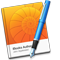# Add and edit equations with LaTeX, MathML, or MathType

You can add mathematical expressions and equations and have them appear in your completed book. To add an equation in LaTeX or MathML format, use iBooks Author, or use MathType if you have MathType 6.7d or later installed.

You can adjust the font size or color of an equation just as you would ordinary text.

Note: Mathematical expressions and equations created with LaTeX, MathML, or MathType can’t be inserted into table cells.

## Add equations with LaTeX or MathML

1. Place the insertion point where you want the equation to appear.

2. Choose Insert > Equation.

3. Enter your LaTeX or MathML equation in the field provided.

When you finish typing, a preview of your equation is displayed.

If the preview shows an empty equation or an error, check your equation.

5. To change the font size or color of a LaTeX or MathML equation, click the equation and adjust the font size and color in the format bar.

6. To edit the equation, double-click it and enter your edits, then click Update.

You can use MathType 6.7d or later to add equations to your book.

Tip: To set MathType as the default equation editing tool, choose iBooks Author > Preferences, click General, and select “Insert and edit equations with MathType.”

1. Place the insertion point where you want the equation to appear.

2. Choose Insert > Equation.

If you set MathType as your default equation editing tool, MathType opens automatically.

3. Enter your equation in the field provided.

See MathType Help for instructions on using the MathType tools.

4. To save your equation, choose File > Close and Return to iBooks Author, then click Yes in the window that appears.

5. To edit the equation, double-click it to open MathType again.

For information on supported LaTeX commands, MathML elements, and MathML attributes, see the Apple Support article iBooks Author: About LaTeX and MathML support.